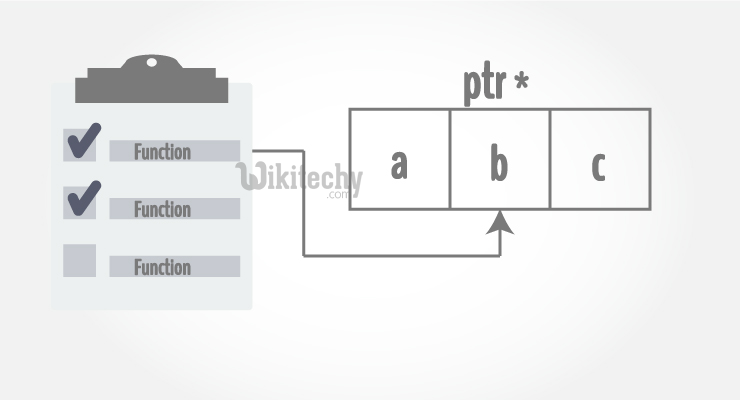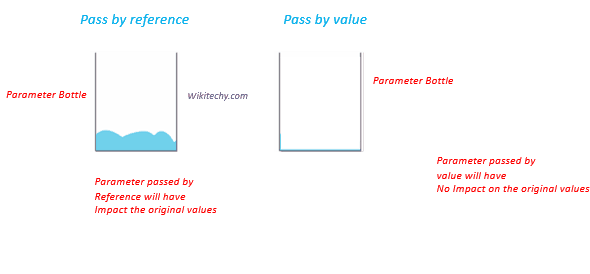# Call by Reference | C++ Call by Reference Using pointers With Examples - Learn C++ - C++ Tutorial - C++ programmingLearn c++ - c++ tutorial - call by reference in c++ - c++ examples - c++ programs

• In this article, you'll learn to pass pointers as an argument to the function, and use it efficiently in your program.• In C++ Functions article, you learned about passing arguments to a function. This method used is called passing by value because the actual value is passed.
• However, there is another way of passing an argument to a function where where the actual value of the argument is not passed. Instead, only the reference to that value is passed.learn c++ tutorials - pass by value vs pass by reference in c++ Example

## Output

• In main(), two integer variables a and b are defined. And those integers are passed to a function swap() by reference.
• Compiler can identify this is pass by reference because function definition is void swap(int& n1, int& n2) (notice the & sign after data type).
• Only the reference (address) of the variables a and b are received in the swap() function and swapping takes place in the original address of the variables.
• In the swap() function, n1 and n2 are formal arguments which are actually same as variables a and b respectively.
• There is another way of doing this same exact task using pointers.

## Example 2: Passing by reference using pointers

• The output of this example is same as before.
• In this case, the address of variable is passed during function call rather than the variable itself.
• Since the address is passed instead of value, dereference operator must be used to access the value stored in that address.
• The *n1 and *n2 gives the value stored at address n1 and n2 respectively.
• Since n1 contains the address of a, anything done to *n1 changes the value of a inmain() function as well. Similarly, b will have same value as *n2.